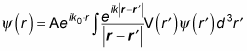##### Quantum Physics For Dummies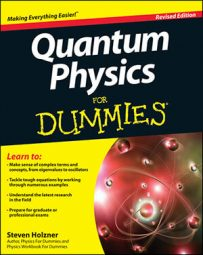The scattering amplitude of spinless particles is crucial to understanding scattering from the quantum physics point of view. To see that, take a look at the current densities, Jinc (the flux density of a given incident particle) and Jsc (the current density for a given scattered particle):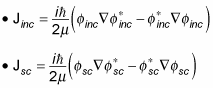(Remember that the asterisk symbol [*] means the complex conjugate. A complex conjugate flips the sign connecting the real and imaginary parts of a complex number.)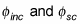into these equations gives you the following, where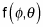is the scattering amplitude: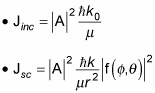Now in terms of the current density, the number of particles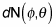scattered into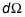and passing through an area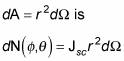Plugging in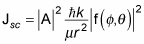into the preceding equation gives you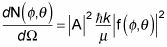Also, recall that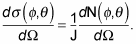You get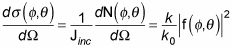And here's the trick — for elastic scattering, k = k0, which means that this is your final result: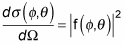The problem of determining the differential cross section breaks down to determining the scattering amplitude.

To find the scattering amplitude — and therefore the differential cross section — of spinless particles, you work on solving the Schrödinger equation: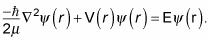You can also write this as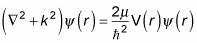You can express the solution to that differential equation as the sum of a homogeneous solution and a particular solution: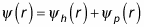The homogeneous solution satisfies this equation: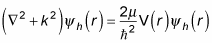And the homogeneous solution is a plane wave — that is, it corresponds to the incident plane wave: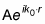To take a look at the scattering that happens, you have to find the particular solution. You can do that in terms of Green's functions, so the solution to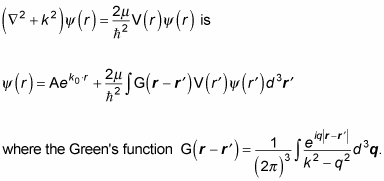This integral breaks down to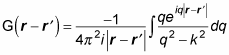You can solve the preceding equation in terms of incoming and/or outgoing waves. Because the scattered particle is an outgoing wave, the Green's function takes this form: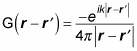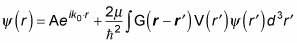So substitutinginto the preceding equation gives you